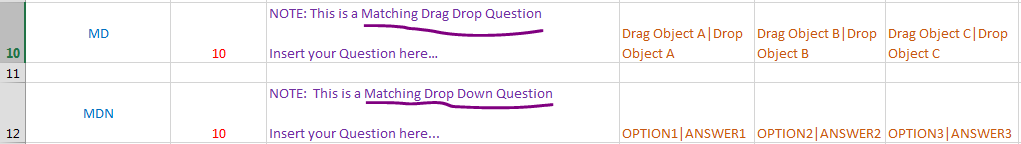# Follow these steps for a successful data import from the Microsoft Excel sheet to Articulate storyline:

Create an Excel sheet with the following columns:

• Quiz Question Type
• Score Points
• Questions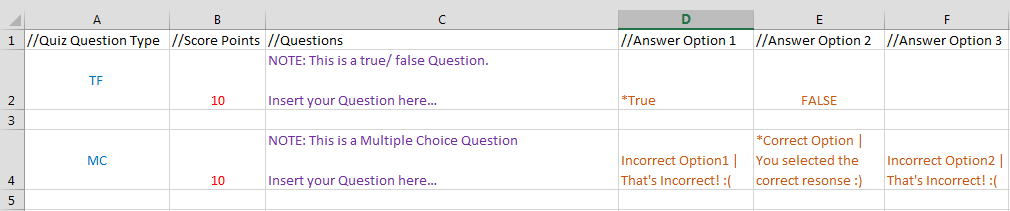In Quiz Question Type we can use abbreviations like:

 Abbreviation Question Type TF True/False MC Multiple Choice MR Multiple Response FIB Fill in the Blank WB Word Bank MD Matching Drag and Drop MDN Matching Drop-down SD Sequence Drag and Drop SDN Sequence Drop-down NUM Numeric LIK Likert PO Pick One PM Pick Many WW Which Word SA Short Answer ESS Essay RD Ranking Drag and Drop RDN Ranking Drop-down HM How Many

Score Pointscolumn is used for graded questions, we can allot marks to correct answers in each quiz. The Points can range from -1000 to 1000. Points for incorrect answers are not supported.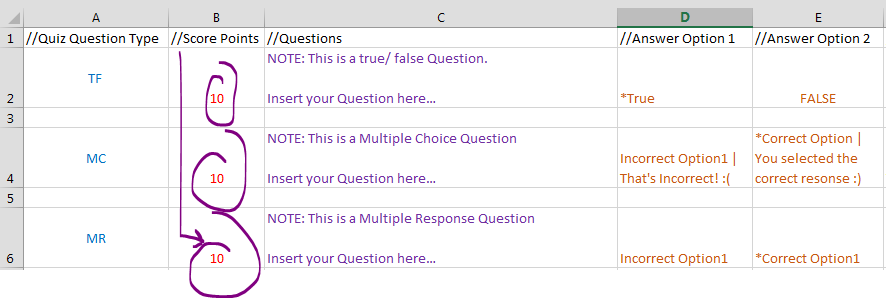Questions Text is required for all questions. In This column you can add your questions as shown below.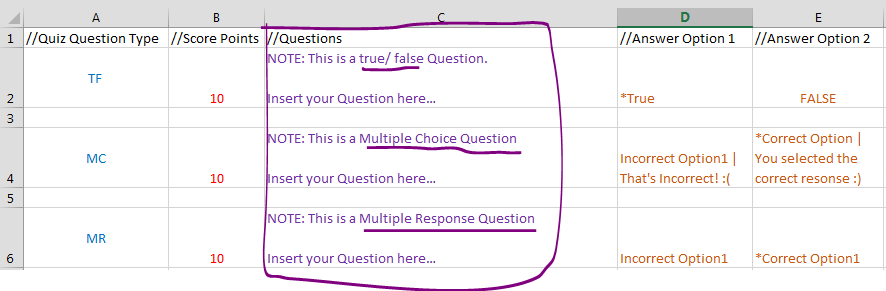You can make upto 10 columns for answer choices and the correct answers are denoted by an Asterisk (*). In the example given below * True and all the underlined options are the correct answers.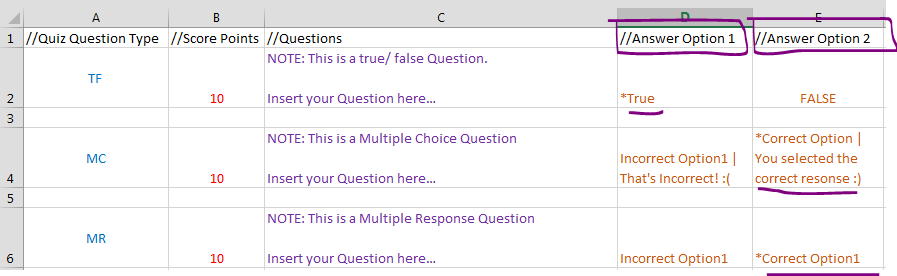For choice-level feedback, separate each answer choice and its feedback with a pipe (|) as shown in the image below.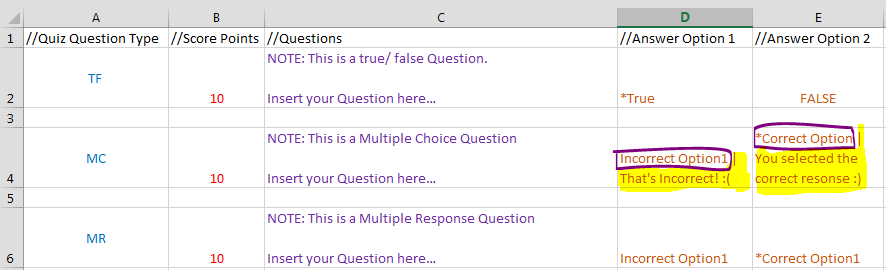For matching questions, separate each answer choice and its match with a pipe (|).